## Monday, 20 January 2014

### xyplot - 'lattice' Package

Lattice is one of the more advanced and popular graphics package available in R. It allows users to create xyplot, density plot and contour maps (2D and 3D) etc. This blog will discuss the use of xyplot() function. This is similar to plot() function but are simpler to code and allows for more complex graphs to be drawn.

To view the built-in examples, use the below code in a similar manner to viewing examples in basic graph functions.

demo(lattice)

The below is an example of simple xyplot.

library(lattice) xyplot(incidence ~ year, melanoma)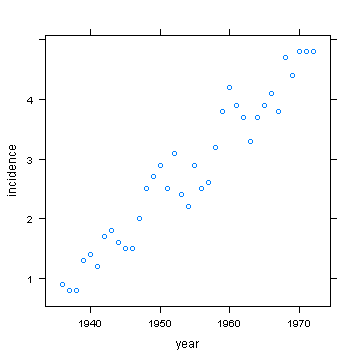To convert the above scatter plot to a different type, we need to assign 'type' parameter as shown below which changes the graph to line graph.

xyplot(incidence ~ year, melanoma, type = "l")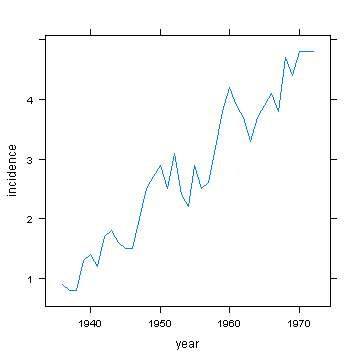There are several options to change 'type'. You can also choose more than one 'type' to superpose different types of graphs. The below example plots regression line through the scatter plot in the first example above.

xyplot(incidence ~ year, melanoma, type = c("p", "r"))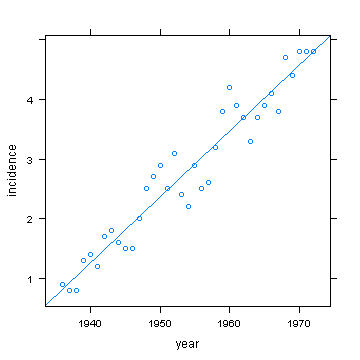The below example inserted a loess line instead of a regression line.

xyplot(incidence ~ year, melanoma, type = c("p", "smooth"))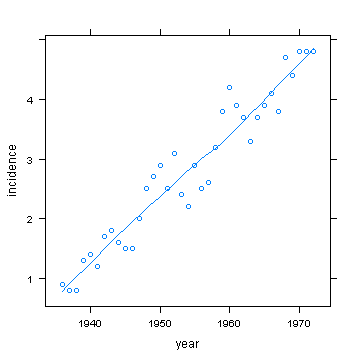The below example is for spline.

xyplot(incidence ~ year, melanoma, type = c("p", "spline"))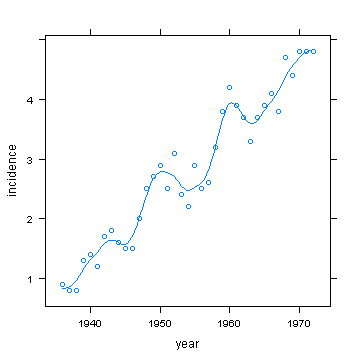The below example shows how to include grid in the background.

xyplot(incidence ~ year, melanoma, type = c("p", "spline", "g"))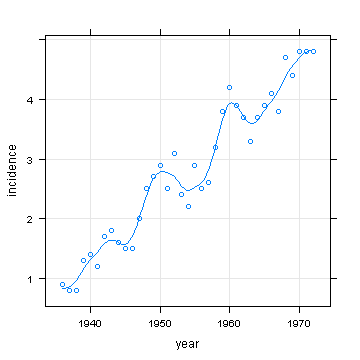Changing the graphic parameters and inserting graph title and subtitle are similar to plot() function. However, to change the format or style of title and subtitle, you need to use par.setting.

xyplot(incidence ~ year, melanoma, type = c("p", "r"), cex = 1.2, pch = 16, col = "red", main = "Melanoma Incidents by Year", sub = "melanoma incidence over time", par.settings = list(par.main.text = list(cex = 1.8, col = "blue"), par.sub.text = list(cex = 1.2, col = "green"), par.xlab.text = list(cex = 0.8, col = "purple"), par.ylab.text = list(cex = 0.8,   col = "steelblue")))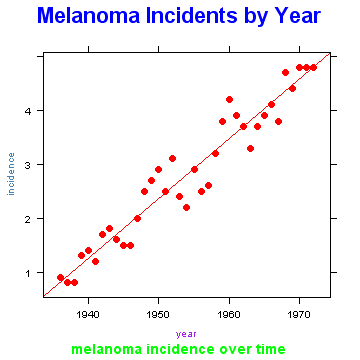The below example shows hoe to display several graphs in multiple panes on the same window. If the variable used for splitting the data into different panes is numeric, it needs to be converted into character format prior to drawing the graph.

xyplot(conc ~ uptake | Type, CO2, type = c("p", "smooth"))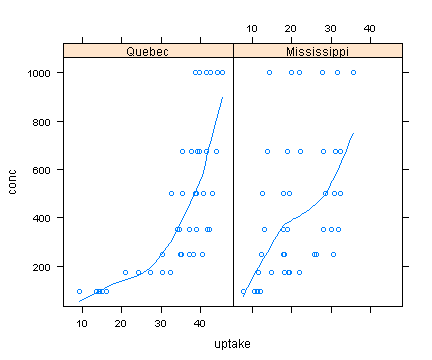The default for using multiple panes fixes the axes to the same scale. To make the axes independent of each other, you can set 'scales' to 'free' as shown below.

xyplot(conc ~ uptake | Type, CO2, type = c("p", "smooth"), scales = "free")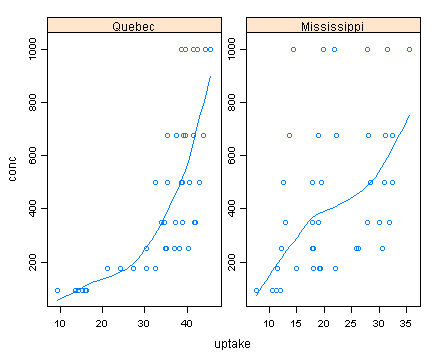If you want to fix one axis but make the other axis 'free', you can assign the code as shown below which fixes y-axis but make x-axis change according to the values in the cell.

xyplot(conc ~ uptake | Type, CO2, type = c("p", "smooth"), scales = list(x = "free"))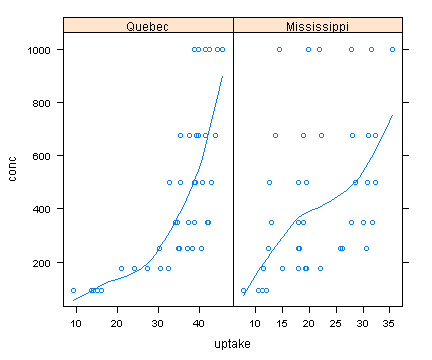To manually assign axis scale, you can assign the code as shown below.

xyplot(conc ~ uptake | Type, CO2, type = c("p", "smooth"), scales = list(x = "free", y = list(limits = c(100, 800))))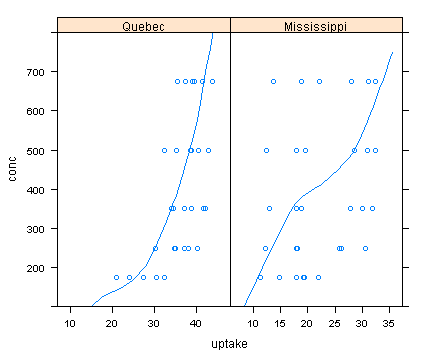To change the layout of the window, assign 'layout' parameter with an appropriate vector as shown below.

xyplot(conc ~ uptake | Type, CO2, type = c("p", "smooth"), scales = "free", layout = c(1, 2))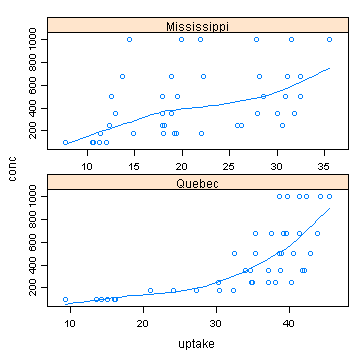To change the size of the text description of pane, use par.strip.text as shown below.

xyplot(conc ~ uptake | Type, CO2, type = c("p", "smooth"), scales = "free", par.strip.text = list(cex = 0.6))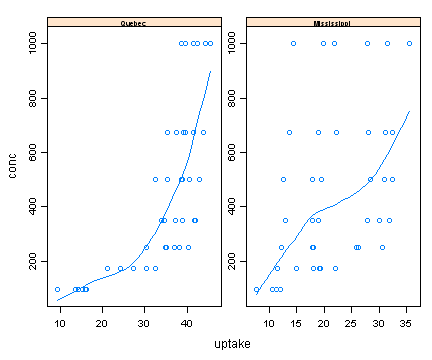The size of the strip of pane, layout.heights need to be changed within par.settings.

xyplot(conc ~ uptake | Type, CO2, type = c("p", "smooth"), scales = "free", par.settings = list(layout.heights = list(strip = 2)))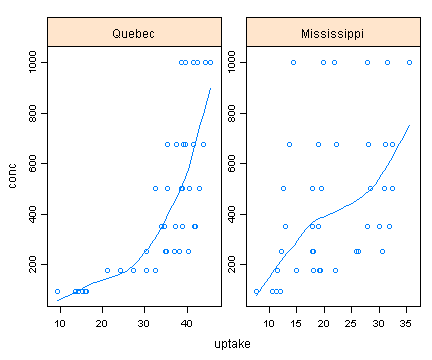The tick mark labels on x & y axis can be changed using 'scales' parameter as shown below.

xyplot(conc ~ uptake | Type, CO2, type = c("p", "smooth"), scales = list(x = list(cex = 0.8, rot = 45), y = list(cex = 1.2, rot = 0)), par.settings = list(layout.heights = list(strip = 2)))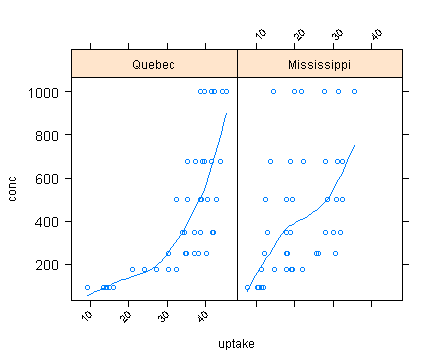To set scale limits:

xyplot(conc ~ uptake | Type, CO2, type = c("p", "smooth"), scales = list(x = list(cex = 0.8, rot = 45), y = list(cex = 1.2, rot = 0, lim = c(0, 500))))

To set different scale limits in each panel:

xyplot(conc ~ uptake | Type, CO2, type = c("p", "smooth"), scales = list(x = list(cex = 0.8, rot = 45), y = list(relation = "free", cex = 1.2, rot = 0, lim = list(c(0, 500), c(0, 1500)))))

The xyplot can categorise data points in relation to the corresponding values of a categorical variable using 'group/groups' parameter.

xyplot(conc ~ uptake | Type, CO2, type = c("p", "smooth"), groups = CO2\$Treatment)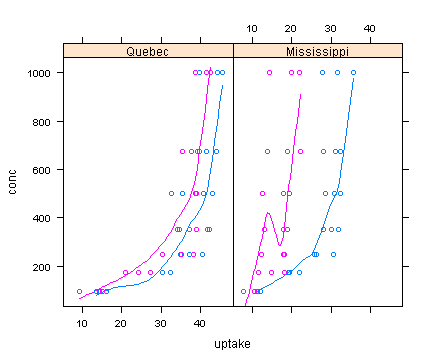The legend that corresponds to the groups can be inserted as shown below.

xyplot(conc ~ uptake | Type, CO2, type = c("p", "smooth"), groups = CO2\$Treatment, auto.key = TRUE)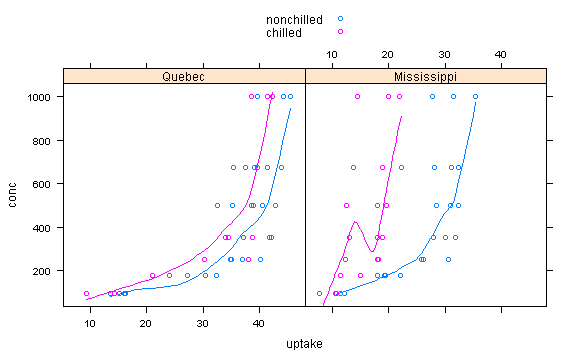The position and contents of legend can be altered as shown below.

xyplot(conc ~ uptake | Type, CO2, type = c("p", "smooth"), pch = 16, groups = CO2\$Treatment, auto.key = list(space = "right", text = c("non-chilled loess", "chilled loess"), cex = 0.7, size = 1.7, lines = TRUE, points = FALSE))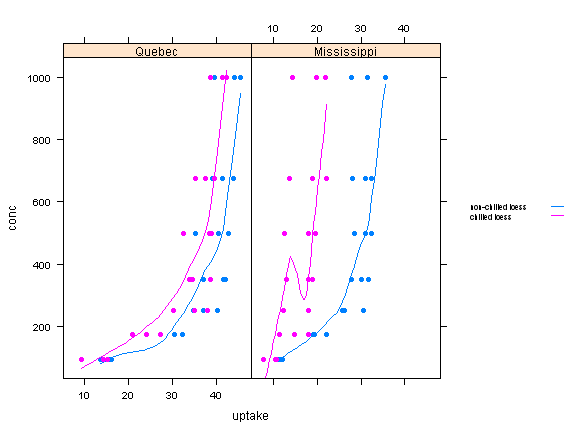When the plotted data points overlap, the transparency of the plots can be changed using 'alpha' parameter. The 'alpha' can be assigned a number in a scale between 0 and 1, with 1 being opaque and 0 being clear.

xyplot(conc ~ uptake | Type, CO2, type = c("p", "smooth"), pch = 16, groups = CO2\$Treatment, alpha = 0.5, auto.key = list(space = "right", text = c("non-chilled loess", "chilled loess"), cex = 0.7, size = 1.7, lines = TRUE, points = FALSE))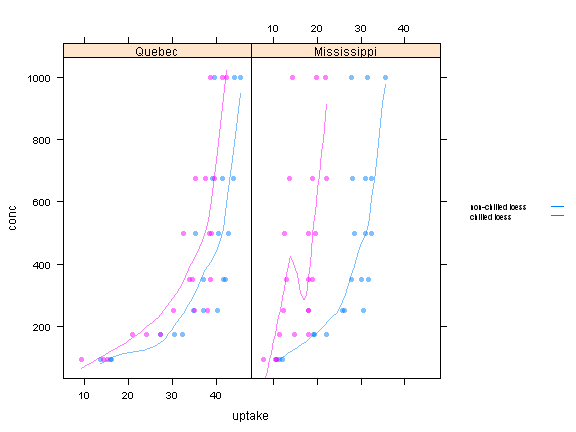To manually assign legend or key:

CO2\$Color<-ifelse(CO2\$Treatment=="chilled","blue","red")

xyplot(conc ~ uptake | Type, CO2, col=CO2\$Color,type="p",pch=16,alpha=0.7,cex=1,
par.settings=list(layout.heights=list(strip=0.8)),par.strip.text=list(cex = 0.6),
key=list(space="right",title="Treatment",cex.title=0.8,points=list(col=c("blue","red"),cex=0.7,pch=16),text=list(lab=c("Chilled","Non-Chilled"),cex=0.7)))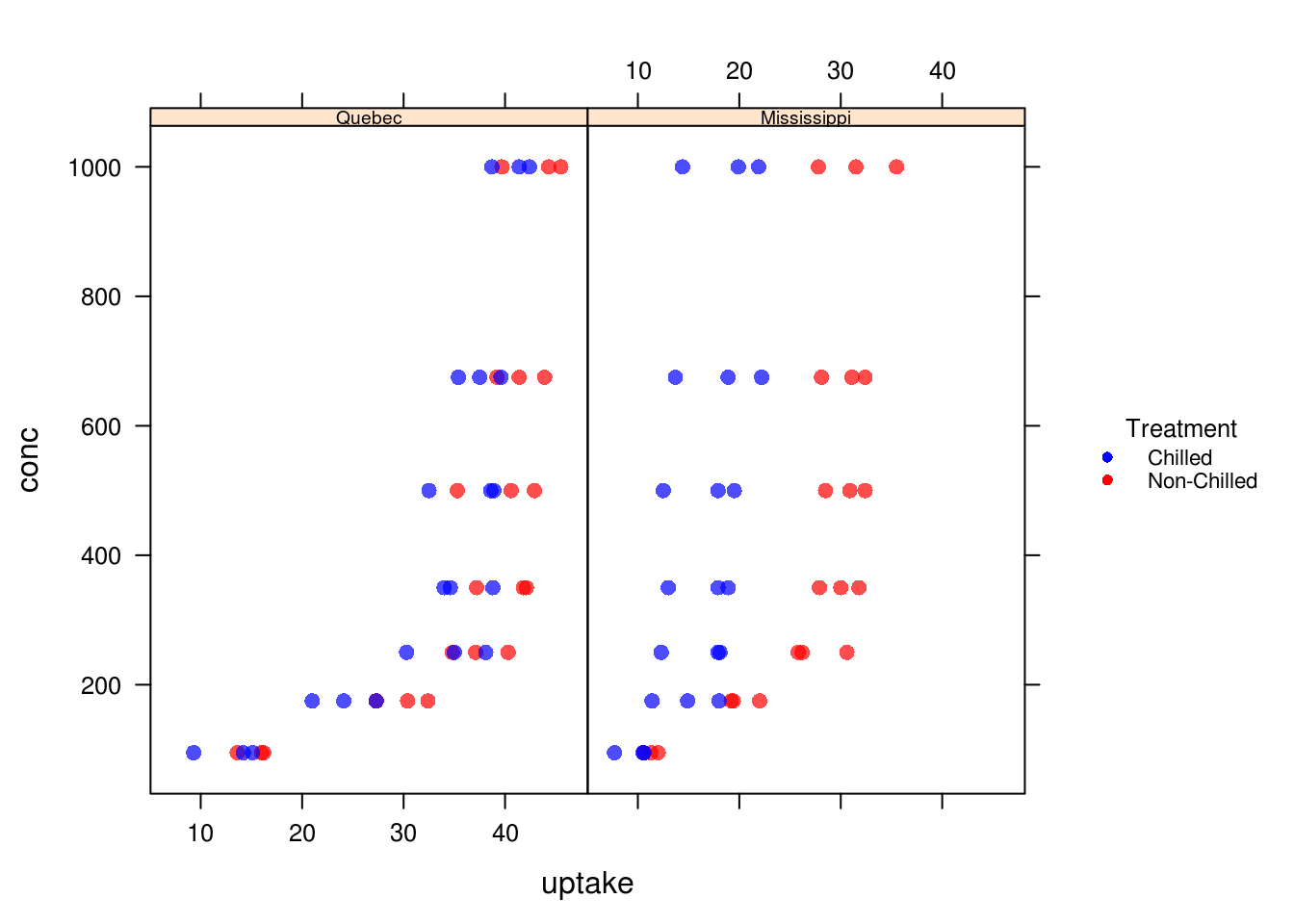To change spacing between key label and key symbol, change the settings for 'between'.

xyplot(conc ~ uptake | Type, CO2, col=CO2\$Color,type="p",pch=16,alpha=0.7,cex=1,
par.settings=list(layout.heights=list(strip=0.8)),par.strip.text=list(cex = 0.6),
key=list(space="right",title="Treatment",cex.title=0.8,
points=list(col=c("blue","red"),cex=0.7,pch=16),text=list(lab=c("Chilled","Non-Chilled"),cex=0.7),between=4))

To change spacing between keys when they are arranged in columns, change settings for 'between.columns'.

g1<-xyplot(conc ~ uptake | Type, CO2, col=CO2\$Color,type="p",pch=16,alpha=0.7,cex=1,main="with column spacing 3",
par.settings=list(layout.heights=list(strip=0.8)),par.strip.text=list(cex = 0.6),
key=list(space="top",column=2,title="Treatment",cex.title=0.8,
points=list(col=c("blue","red"),cex=0.7,pch=16),text=list(lab=c("Chilled","Non-Chilled"),cex=0.7),between=2,between.columns=3))

g2<-xyplot(conc ~ uptake | Type, CO2, col=CO2\$Color,type="p",pch=16,alpha=0.7,cex=1,main="with column spacing 8",
par.settings=list(layout.heights=list(strip=0.8)),par.strip.text=list(cex = 0.6),
key=list(space="top",column=2,title="Treatment",cex.title=0.8,
points=list(col=c("blue","red"),cex=0.7,pch=16),text=list(lab=c("Chilled","Non-Chilled"),cex=0.7),between=2,between.columns=8))

grid.arrange(g1,g2,ncol=2)

There are many other options to alter graphic parameters used by xyplot. To see these options, use the below command to list the parameters.

trellis.par.get()

When multiple pane is used, the graphic parameters within each pane could be controlled or additional graphs inserted or superposed.

To include 'abline' within each pane, 'panel' function can be used to insert the straight lines as shown below.

Dat <- split(CO2, CO2\$Plant) xyplot(conc ~ uptake | Type, CO2, type = "p", groups = CO2\$Treatment, pch = 16, alpha = 0.5, panel = function(x, y, ...) { panel.xyplot(x, y, ...) panel.abline(v = 30, lty = 3, col = "red") })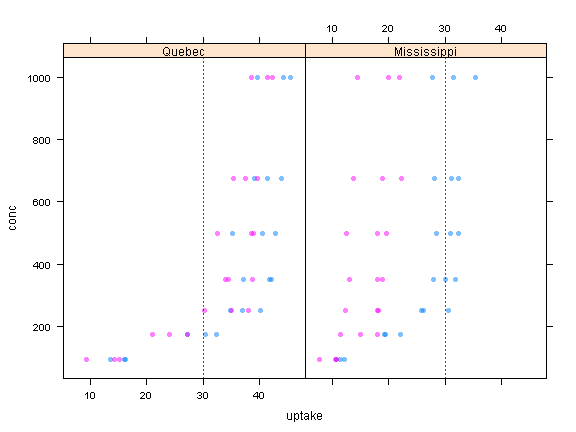To format the plots or lines in relation to corresponding values of a reference variable, 'subscript' can be used. If the reference variable is quoted as in a single pane graph, the values will not align correctly between the plotted variable and the reference variable.

xyplot(conc ~ uptake | Type, CO2, type = "p", K = CO2\$Treatment, groups = CO2\$Plant, panel = function(x, y, K, groups, ..., subscripts) { panel.xyplot(x, y, pch = 16, col = groups, cex = ifelse(K[subscripts] == "chilled", 2, 1), alpha = 0.5) panel.abline(v = 30, lty = 3, col = "red") })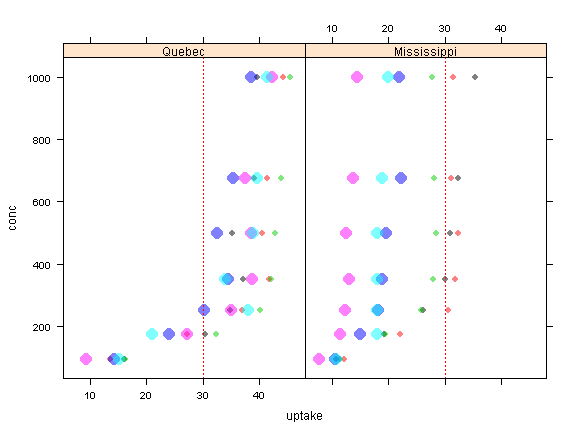For functions related to styling and formatting of strip label, use below.

xyplot(conc ~ uptake | Type, CO2, type = "p", K = CO2\$Treatment, groups = CO2\$Plant,
par.settings=list(strip.background=list(col="ivory"),layout.widths=list(strip.left=5)),
par.strip.text=list(col="brown"),layout=c(1,2),
strip=FALSE,strip.left=strip.custom(horizontal=TRUE),
panel = function(x, y, K, groups, ..., subscripts) { panel.xyplot(x, y, pch = 16, col = groups, cex = ifelse(K[subscripts] == "chilled", 2, 1), alpha = 0.5) panel.abline(v = 30, lty = 3, col = "red") })

To control gap between panels, use below.

xyplot(conc ~ uptake | Type, CO2, type = "p", K = CO2\$Treatment, groups = CO2\$Plant,
par.settings=list(strip.background=list(col="ivory"),layout.widths=list(strip.left=5)),
par.strip.text=list(col="brown"),layout=c(1,2),
strip=FALSE,strip.left=strip.custom(horizontal=TRUE),
between=list(y=0.5),
panel = function(x, y, K, groups, ..., subscripts) { panel.xyplot(x, y, pch = 16, col = groups, cex = ifelse(K[subscripts] == "chilled", 2, 1), alpha = 0.5) panel.abline(v = 30, lty = 3, col = "red") })

To change the scale of axis, use 'at' parameter within 'scale' parameter e.g. scale=list(x=list(at=...))

For example, the below is the default x-axis scale of xyplot.

xyplot(shape~peri,rock,xlab="peri",ylab="shape",col="maroon2",type="p",
cex=1.5,main="data: rock",pch=16)

and, the below is the same xyplot with smaller units (500) assigned for x-axis.

xyplot(shape~peri,rock,xlab="peri",ylab="shape",col="maroon2",type="p",
cex=1.5,main="data: rock",pch=16,
scale=list(x=list(at=seq(0,5000,500))))

If you want to draw xyplot without axis, use draw=FALSE inside 'scale' parameter.

Dat<-as.data.frame(cbind(sin(seq(-pi,pi,length=100)),cos(seq(-pi,pi,length=100))))
xyplot(V2~V1,Dat,type="l",col=rgb(255,0,0,max=255),
scale=list(draw=FALSE),xlab="",ylab="")

If you want to remove the frame around the graph, control axis.line within 'par.settings'.

xyplot(V2~V1,Dat,type="l",col=rgb(255,0,0,max=255),
scale=list(draw=FALSE),xlab="",ylab="",par.settings=list(axis.line=list(col=0)))

To remove axis lines and only show tick marks and labels on bottom and left sides, you need to first remove axis line by setting the colour to either "transparent" or same as background colour, then use axis function to identify sides to draw ticks.

xyplot(shape~peri,rock,xlab="peri",ylab="shape",col="maroon2",type="p",cex=1.5,
main="data: rock",pch=16,par.settings=list(axis.line=list(col="transparent")),
axis=function(side,line.col,...){
if(side%in%c("left","bottom")){
axis.default(side=side,ticks="yes",line.col="black",...)
}
})

#### 1 comment:

1.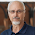Much appreciated. Excellent set of examples for learning Trellis graphics. Thank you for posting.